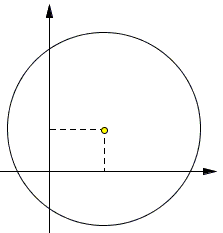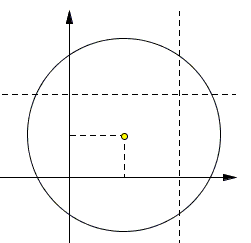# Areas In CircleThe point P = P(a, b) is located in the first quadrant. Consider a circle centered at the point P with radius greater than a² + b², and denote the area of the part of this circle located in the i-th quadrant (i = 1, 2, 3, 4) by Si . Find S1 - S2 + S3 - S4.

This problem has been included in the 2009-2010 international online mathematical olympiad run by the Ariel University Center in Samaria (Israel).

SolutionThe point P = P(a, b) is located in the first quadrant. Consider a circle centered at the point P with radius greater than a² + b², and denote the area of the part of this circle located in the i-th quadrant (i = 1, 2, 3, 4) by Si . Find S1 - S2 + S3 - S4.

### Solution

Draw two extra lines, x = 2a and y = 2b:These two lines together with the two axes, split the area of the circle into 9 parts, eight of which cancel out in S1 - S2 + S3 - S4. The only remaining one is the central rectangle whose area is 2a·2b = 4ab.